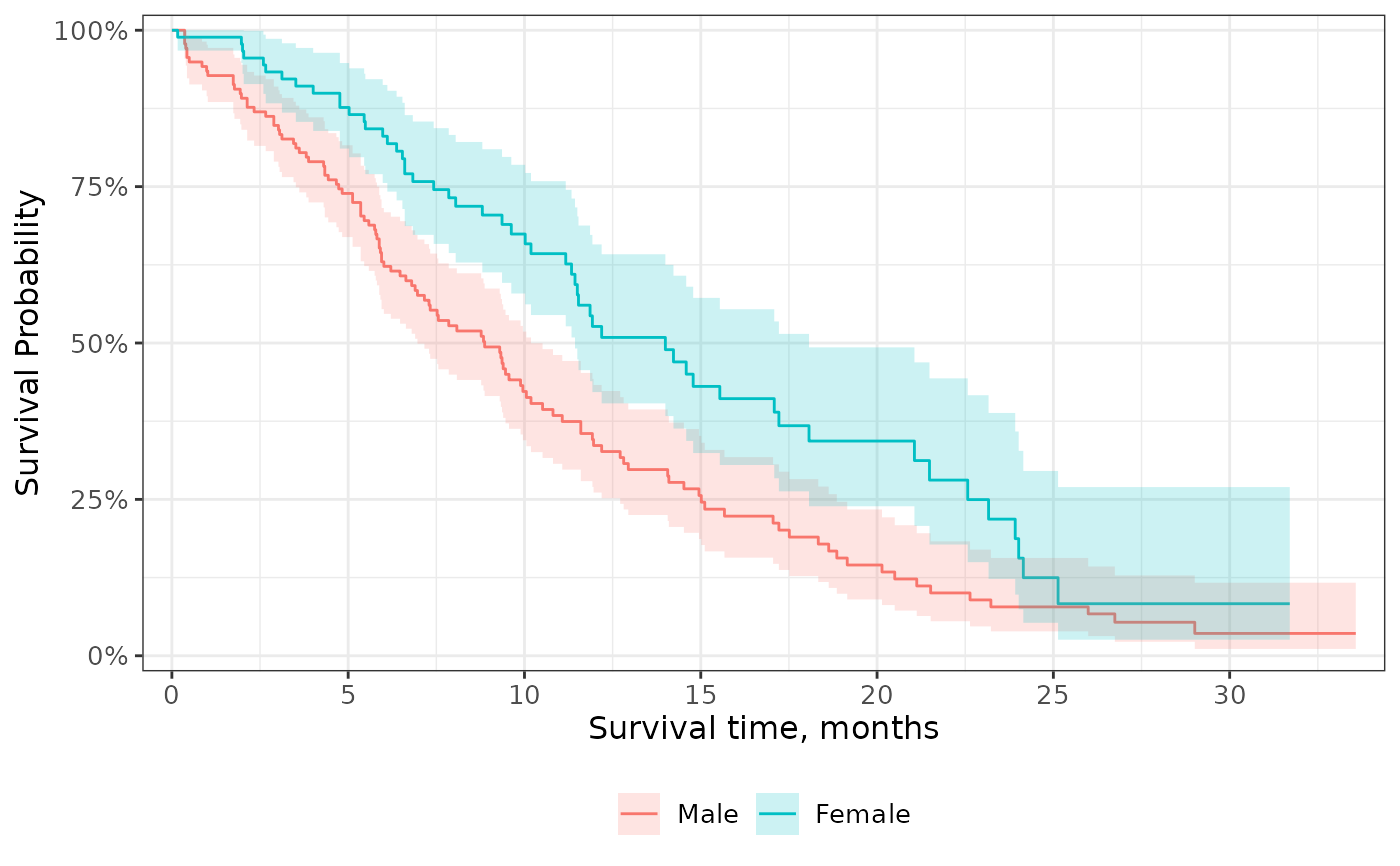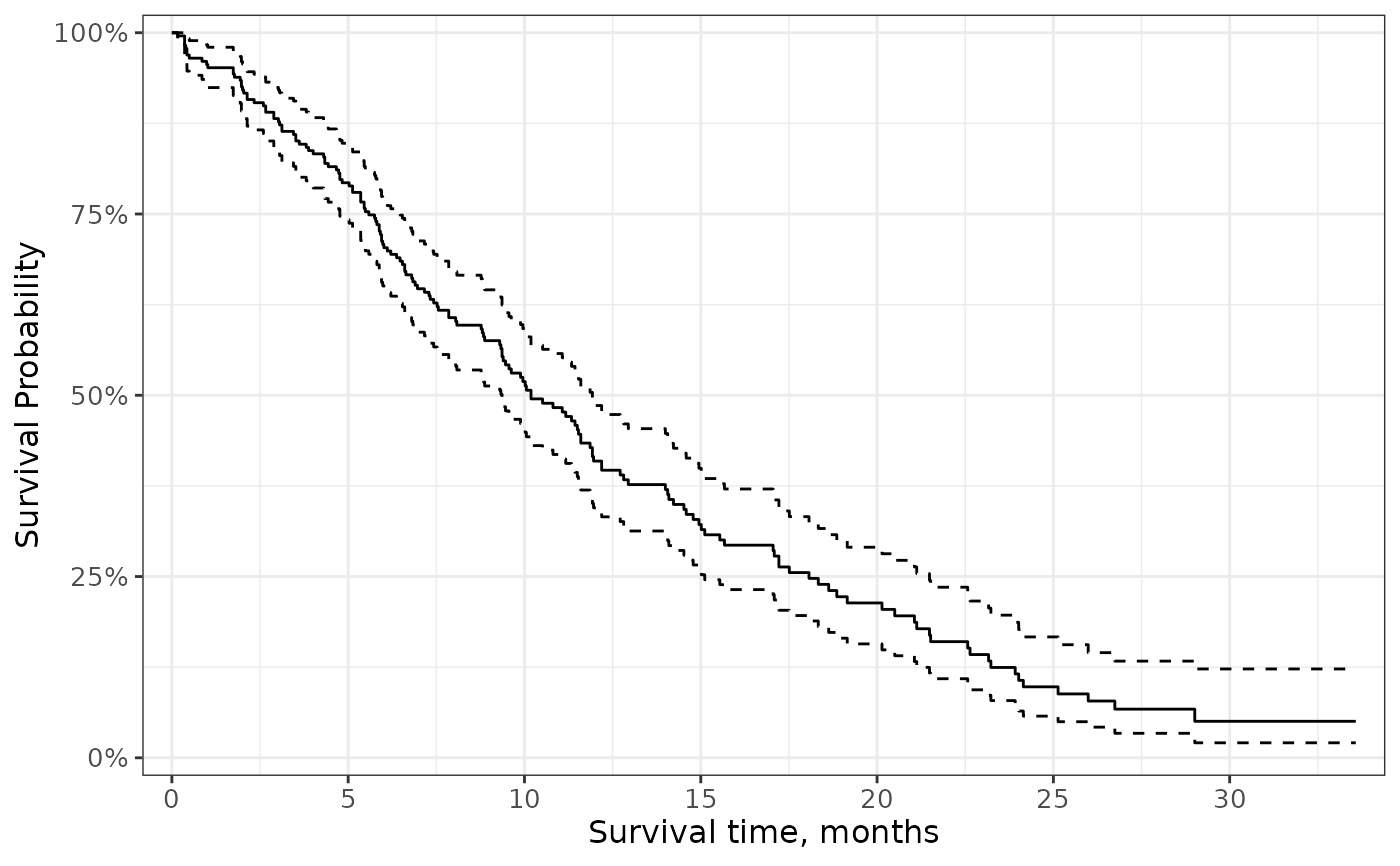Add a confidence interval represented by either a ribbon or lines.

## Usage

add_confidence_interval(type = c("ribbon", "lines"), ...)

## Arguments

type

string indicating the type of confidence interval to draw. Must be one of c("ribbon", "lines")

...

arguments pass to geom.

• type = 'ribbon': Defaults are ggplot2::geom_ribbon(alpha = 0.2, color = NA, ...)

• type = 'lines': Defaults are ggplot2::geom_step(linetype = "dashed", na.rm = TRUE, ...)

## Value

a ggplot2 figure

Visit the gallery for examples modifying the default figures

## Examples

survfit2(Surv(time, status) ~ sex, data = df_lung) %>%
ggsurvfit() +